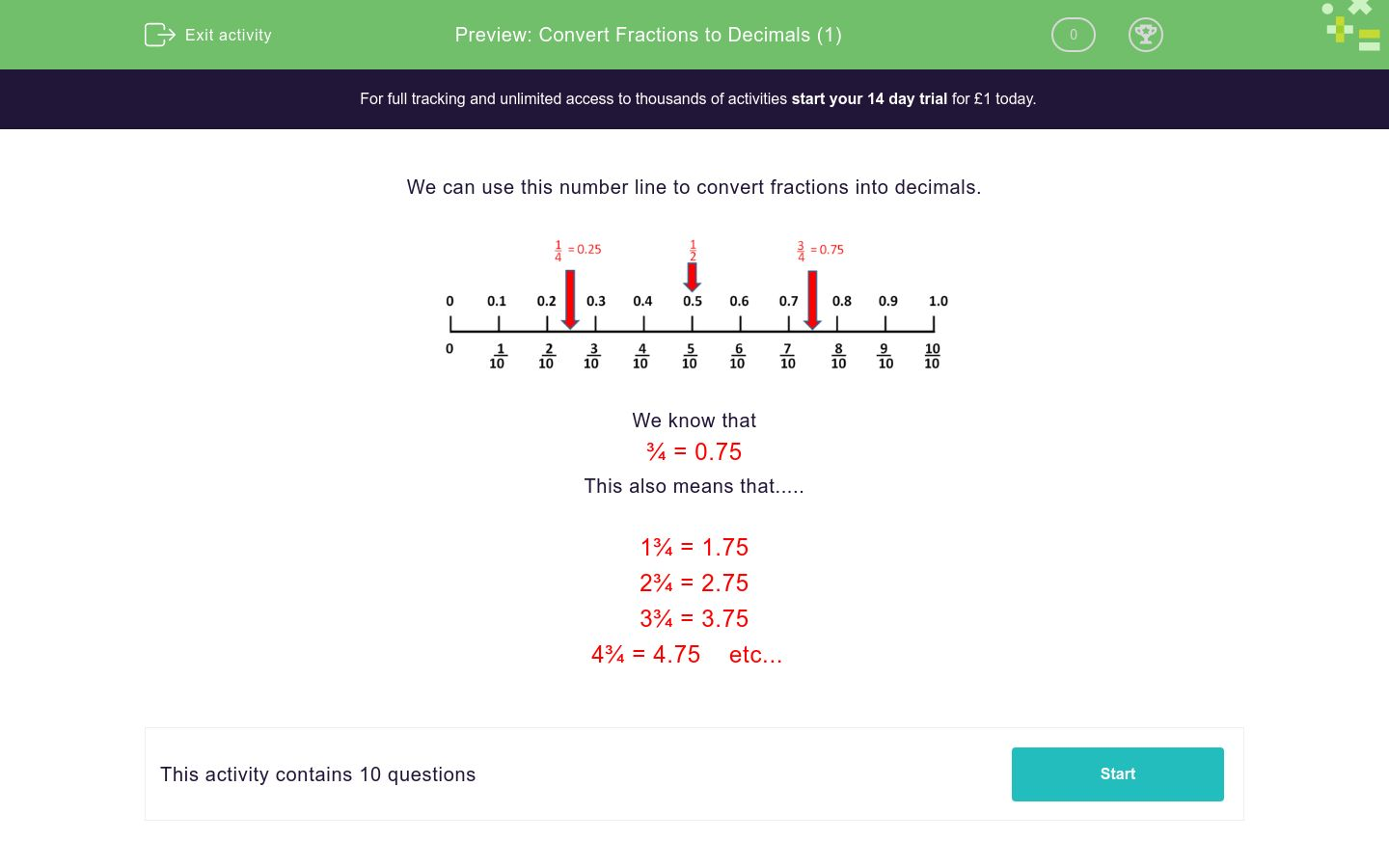# Convert Fractions to Decimals (1)

In this worksheet, students convert simple mixed numbers into decimals.Key stage:  KS 2

Curriculum topic:   Number: Fractions and Decimals

Curriculum subtopic:   Recognise Decimal Equivalents

Difficulty level:### QUESTION 1 of 10

We can use this number line to convert fractions into decimals.We know that

¾ = 0.75

This also means that.....

1¾ = 1.75

2¾ = 2.75

3¾ = 3.75

4¾ = 4.75    etc...

---- OR ----